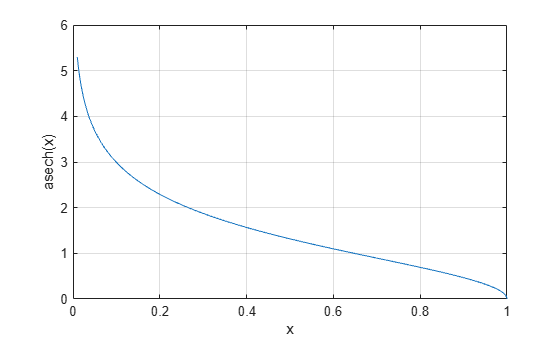# asech

Inverse hyperbolic secant

## Syntax

``Y = asech(X)``

## Description

example

````Y = asech(X)` returns the inverse hyperbolic secant of the elements of `X`. The function accepts both real and complex inputs. All angles are in radians.```

## Examples

collapse all

Find the inverse hyperbolic secant of the elements of vector `X`. The `asech` function acts on `X` element-wise.

```X = [2 -3 1+2i]; Y = asech(X)```
```Y = 1×3 complex 0.0000 + 1.0472i 0.0000 + 1.9106i 0.3966 - 1.3845i ```

Plot the inverse hyperbolic secant function over the interval $0.01\le x\le 1$.

```x = 0.01:0.001:1; plot(x,asech(x)) grid on xlabel('x') ylabel('asech(x)')```## Input Arguments

collapse all

Hyperbolic secant of angle, specified as a scalar, vector, matrix, or multidimensional array. The `asech` operation is element-wise when `X` is nonscalar.

Data Types: `single` | `double`
Complex Number Support: Yes

collapse all

### Inverse Hyperbolic Secant

For real values $x$ in the domain $0, the inverse hyperbolic secant satisfies

`${\text{sech}}^{-1}\left(x\right)={\mathrm{cosh}}^{-1}\left(\frac{1}{x}\right)=\mathrm{log}\left(\frac{1}{x}+\sqrt{\frac{1}{{x}^{2}}-1}\right).$`

For complex numbers $z=x+iy$ as well as real values in the regions $-\text{\hspace{0.17em}}\infty and $1\le z<\infty$, the call `asech(z)` returns complex results.